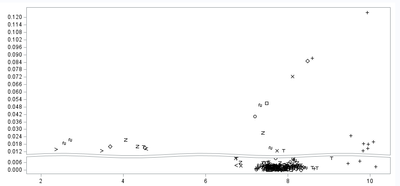## SGPLOT, two Different Ranges on Y Axis, with different Scales for Each

I am doing a scatter plot on which most of the values are in a narrow lower band on the X axis.  I would like to show that lower, narrow band (with its dense data points) at a more focused scale (i.e., wider data points on Y axis), while compressing the  rest of the Y axis since there's not as much there.

It looks like this now:I would like that narrow lower section to be tall, and the upper section correspondingly short.  Is that doable?  Otherwise there's really no point for me to split it at all.

Here is my current code:

``````proc sgplot data = fpepc0 dattrmap = atmap;
scatter x = pclog y = fpe / name   = "scatter1"
group  = avisitn groupdisplay = cluster
attrid = atrid;
xaxis label         = 'Log, Plasma Concentration (ng/mL)'
labelattrs    = (family = "courier new" size = 9.9 pt color = black weight = bold)
offsetmin     =  .04
offsetmax     =  .04;
yaxis label         = 'FPE Rate Constant (1/min)'
ranges        = (0 - 0.010 0.011-0.125)
labelattrs    = (family = "courier new" size = 9.9 pt color = black weight = bold);

format avisitn visf. ;

run; ``````

Thank you, experts...

3 REPLIES 3

## Re: SGPLOT, two Different Ranges on Y Axis, with different Scales for Each

Data would be nice and since you are using a Dattrmap data set then that data set as well.

You range of values is so close that , i.e. making a "break" that is all of 0.001 "units" wide not much use there.

I might be tempted to add another variable for the lower and upper ranges and then use SGPANEL with Panelby using that variable and setting the Uniscale=Column so the "row" ranges could vary between the panels.

With the appropriate added variable something like this would create two panels with approximately equal amount of Y axis for the range of values.  The value of the lower range verticalgroupvariable should be larger than for the top group so that it is placed second in the graph stack. The noheader option supresses have the name and value of the verticalgroupvarible appear at the top of each panel.

```proc SGPANNEL data = fpepc0 dattrmap = atmap;
Panelyby verticalgroupvariable / uniscale=column columns=1 noheader;
scatter x = pclog y = fpe / name   = "scatter1"
group  = avisitn groupdisplay = cluster
attrid = atrid;
xaxis label         = 'Log, Plasma Concentration (ng/mL)'
labelattrs    = (family = "courier new" size = 9.9 pt color = black weight = bold)
offsetmin     =  .04
offsetmax     =  .04;
yaxis label         = 'FPE Rate Constant (1/min)'

labelattrs    = (family = "courier new" size = 9.9 pt color = black weight = bold);

format avisitn visf. ;

run; ```

## Re: SGPLOT, two Different Ranges on Y Axis, with different Scales for Each

Thank you, Mr. Ballard.  I will think about that and give it a shot.

Yeah, I know what you mean about not really having enough "spread" in the values to give us a reason to do this.  I just asked the requestors about that a few minutes ago.

Thank you again, you always come through for me..Ksharp
Super User

## Re: SGPLOT, two Different Ranges on Y Axis, with different Scales for Each

/*
Usine LOG scale for Y axis,
if you have extreme obs of Y .
*/
proc sgplot data=sashelp.heart ;
scatter x=height y= weight;
yaxis type=log logbase=10;
run;
Discussion stats
• 3 replies
• 202 views
• 3 likes
• 3 in conversation